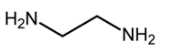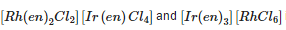# Coordination Compounds Practice Quiz - 1

## 25 Questions MCQ Test Additional Question Bank | Coordination Compounds Practice Quiz - 1

Description
Attempt Coordination Compounds Practice Quiz - 1 | 25 questions in 50 minutes | Mock test for Class 9 preparation | Free important questions MCQ to study Additional Question Bank for Class 9 Exam | Download free PDF with solutions
QUESTION: 1

### Ligand en is an example of

Solution:QUESTION: 2

### A group of atoms can function as a ligand only when

Solution:

Ligands are electron donors.

QUESTION: 3

### The oxidation number of Fe in K4[Fe(CN)6] is

Solution:

CN has charge of –1 charge.

QUESTION: 4

The coordination number of the central ion may be obtained from

Solution:

Coordination number of the central ion is equal to no. of coordination bonds formed with surrounding ions.

QUESTION: 5

In which of the following compounds the oxidation state of the nickel atom is 0?

Solution:

CO is neutral ligand so Ni has 0 oxidation state.

QUESTION: 6

What is the coordination number of chromium in [Cr(NH3)2(H2O2)2]Cl3?

Solution:

There are 4 ligands attachted so CN = 4.

QUESTION: 7

Which of the following is non – ionizable?

Solution:

In A there is no ion present outside the coordination sphere so it is ionizable.

QUESTION: 8

Which of the following pair contains complex salt and double salt respectively?

Solution:

[Cu(NH3)4]SOthis is complex salt while
K2SO4Al2(SO4)3.24H2O is double salt.

QUESTION: 9

In which of the following compounds, the metal is in the lowest oxidation state?

Solution:

CO is neutral so Mn has zero oxidation state.

QUESTION: 10

Effective atomic number of Fe in the complex K4[Fe(CN)6] is

Solution:

26+2(6)-2=36

QUESTION: 11

The correct IUPAC name of [Fe(C5H5)2] is

Solution:

C5H5 is cyclopentadienyl ligand. Name of ligand is written first.

QUESTION: 12

Which of the following species is expected to be colourless?

Solution:

Ti is in d0 state so no d - d transition.

QUESTION: 13

The correct IUPAC name of K2[Zn(OH)4] is

Solution:

OH is hydroxo ligand. Name of ligand is named first.

QUESTION: 14

Lithiumtetrahydridoaluminate is represented as

Solution:

Lithiumtetrahydridoaluminate is Li[AlH4]

QUESTION: 15

The correct name of the compound [Cu(NH3)4](NO3)is

Solution:

Tetraamminecopper(II)nitrate is [Cu(NH3)4](NO3)

QUESTION: 16

Which of the following compound would exhibit coordination isomerism?

Solution:

Coordination isomerism is exhibited the the compounds in which cationic and anionic species is coordination complex.

QUESTION: 17

The kind of isomerism exhibited byis

Solution:

There is exchange of ligands between the cationic and anion species in coordination sphere.

QUESTION: 18

The complex with highest stability constant at 298K is

Solution:

Stability is proportional to CFSE value which is dependent upon Δo value. CN- is stronger field which increases the CFSE.

QUESTION: 19

The tetrahedral complex, bis(benzoylacetonato)beryllium(II) will show

Solution:

This complex is non superimposable mirror image.

QUESTION: 20

What is used as the complexing agent in volumetric analysis of ions like Mg2+ and Ca2+

Solution:

EDTA is hexadentate ligand so it forms stable chelate complex with metal ions.

QUESTION: 21

Which of the following cation does not form an ammine complex with excess of ammonia?

Solution:

Nais s block element so doesnot form ammine complex.

QUESTION: 22

Blue solution of CuSO4 becomes darker when treated with ammonia because

Solution:

Ammonia forms stable complex with Cu2+

QUESTION: 23

According to Werner’s theory, the secondary valences of the central atom correspond to its

Solution:

According to Werner’s theory CN is equal to secondary valency.

QUESTION: 24

Tetraaminecopper(II) ion is a square planar complex with one unpaired electron. According to valence bond theory the hybrid state of copper should be

Solution:

Tetraaminecopper(II) ion is square planar so hybridization is dsp2

QUESTION: 25

The IUPAC name of KAl(SO4)2.12H20 is

Solution:

This is double salt so name is written in sequential manner.Use Code STAYHOME200 and get INR 200 additional OFF Use Coupon Code